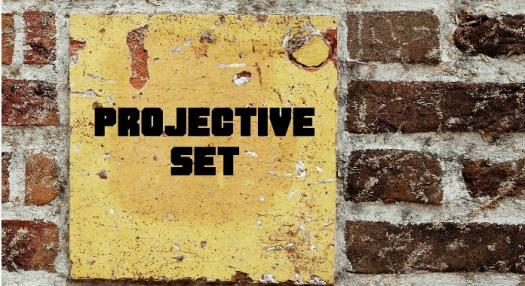10 Questions | Total Attempts: 103SettingsThe projective card game is a real-time card game derived from an older version of the game called the set. With this game, the deck contains cards consisting of colored dots, where players are expected to attempt to find sets among these cards. What do you know about this card game? Take our quiz and find out now.

• 1.
How many binary attributes does the game have?
• A.

6

• B.

4

• C.

5

• D.

3

• 2.
What's the other term for binary attributes?
• A.

Wits

• B.

Rits

• C.

Tits

• D.

Bits

• 3.
How are tits represented?
• A.

By colored dots

• B.

By red dots

• C.

By blue dots

• D.

By yellow dots

• 4.
How many cards are there in the game?
• A.

50

• B.

53

• C.

63

• D.

60

• 5.
How many cards are laid on the table in the original version of the game?
• A.

10

• B.

12

• C.

14

• D.

20

• 6.
When does the game end?
• A.

When  the deck is depleted

• B.

When players are tired

• C.

When there's only one player left

• D.

When 1 player makes the biggest combination

• 7.
What's the rule in the 7 card version?
• A.

It allows sets of small size rather than just sets of size three

• B.

It allows sets of medium size rather than just sets of size three

• C.

It allows sets of any size rather than just sets of size three

• D.

It allows sets of large size rather than just sets of size three

• 8.
What is the collection of vectors in the game?
• A.

It's the finite projective space with order 3 dimension 5

• B.

It's the finite projective space with order 1 dimension 5

• C.

It's the finite projective space with order 2 dimension 5

• D.

It's the finite projective space with order 2 dimension 10

• 9.
How many cards can exist in a set?
• A.

15 out of 81

• B.

25 out of 81

• C.

20 out of 81

• D.

20 out of 100

• 10.
In a projective set, how many cards can exist?
• A.

32 out of 63

• B.

30 out of 60

• C.

22 out of 53

• D.

12 out of 43

Related TopicsBack to top